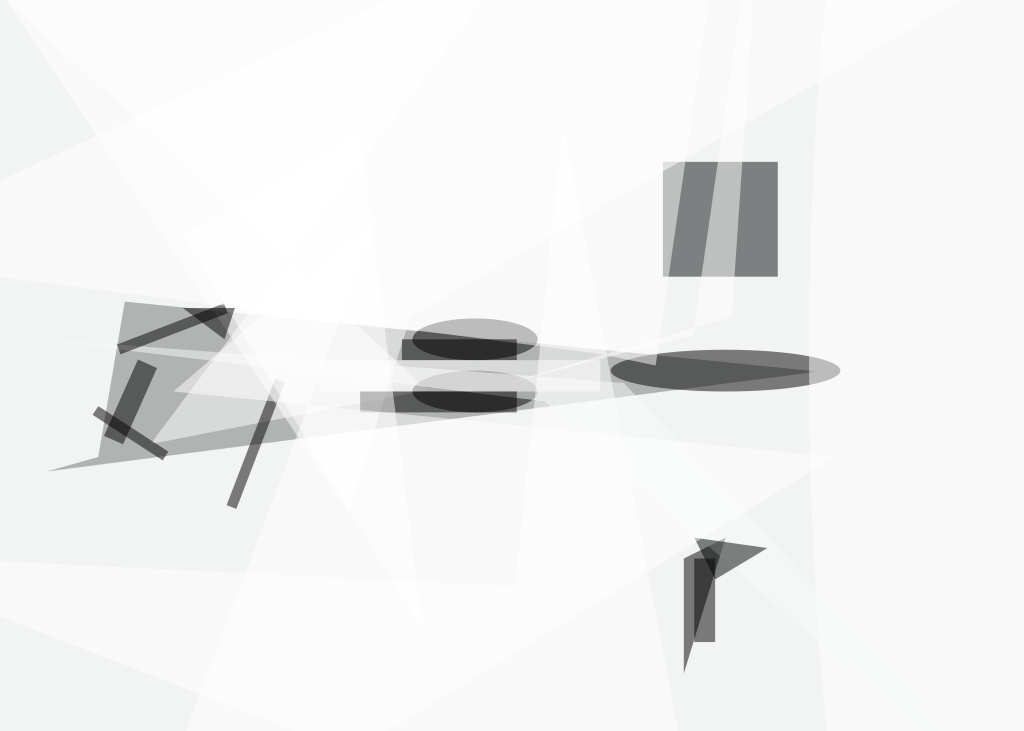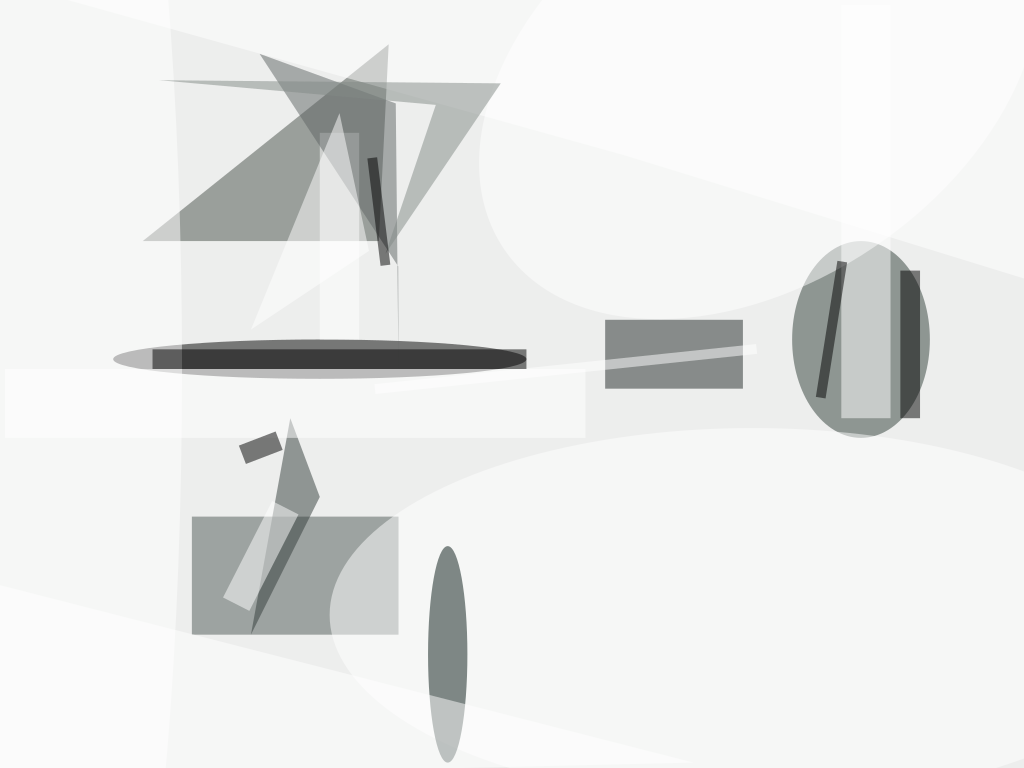Equations have been derived to describe the deflection of a circular membrane under both in-plane and transverse loads. More specifically, the equations describe the radial (in-plane) and perpendicular-to-the-plane deflections for the case of a circular membrane that has been stretched at its periphery with a uniform preload to keep it taut, then clamped rigidly at its periphery, and then subjected to differential pressure.

Equations for deflection of a rigidly clamped circular membrane with differential pressure but without pre-stretching were presented in "Deflection of Circular Membrane Under Differential Pressure" (GSC-13783), NASA Tech Briefs, Vol. 22, No. 5 (May 1998), page 78. The present equations are generalized versions of the previously reported equations. As before, the derivation follows a strain-energy/virtual deflection approach, which is common in stress-and-strain problems of this kind. The departure from the previously reported equations involves the incorporation of additional terms to account for the change in strain energy associated with work done by the preload.

The displacements of the membrane under load are described by the equations

1.and

2.where r is the radial coordinate, a is the radius of the clamping edge, w is the transverse displacement (that is, the deflection perpendicular to the nominal membrane plane) at radius r, w0 is the maximum transverse displacement, u is the radial displacement at radius r, c1 and c2 are constants, p is the preload force, is Poisson's ratio of the membrane material, E is the Young's modulus of the membrane material, and h is the thickness of the membrane. The term p(1–v)r/2πaEh in equation 2 describes the component of radial displacement attributable to the preload.

The radial and transverse strains are given, respectively, by

1.and

2.The strain energy associated with stretching of the membrane is given by

1.To calculate the deflection of the membrane, one must solve the foregoing equations to find c1, c2, and w0. First, one substitutes the right sides of equations (1) through (4) for the corresponding terms in equation 5. Using the resulting form of equation (5), one finds c1 and c2 by imposing the requirements that

1.and

2.Next, one imposes the requirement that the change in work done by the differential pressure acting through a virtual displacement equal the change in strain energy associated with the virtual displacement. If the virtual displacement is chosen to be δw δw0, then this requirement is expressed by the equation

1.where q is the differential pressure on the membrane. Eventually, one obtains the following equations:

1.where

2.Equation 9 can readily be solved to obtain the maximum deflection, w0, at the center of the membrane.

This work was done by Alfonso Hermida of Goddard Space Flight Center. GSC-14223##### NASA Tech Briefs Magazine

This article first appeared in the September, 1999 issue of NASA Tech Briefs Magazine.

Read more articles from the archives here.